×#### Thank you for registering.

One of our academic counsellors will contact you within 1 working day.

Click to Chat

1800-1023-196

+91-120-4616500

CART 0

• 0

MY CART (5)

Use Coupon: CART20 and get 20% off on all online Study Material

ITEM
DETAILS
MRP
DISCOUNT
FINAL PRICE
Total Price: Rs.

There are no items in this cart.
Continue Shopping• Complete JEE Main/Advanced Course and Test Series
• OFFERED PRICE: Rs. 15,900
• View Details

```Chapter 9: Triangle and its Angles Exercise – 9.1

Question: 1

In a ΔABC, if ∠A = 55°, ∠B = 40°, Find ∠C.

Solution:

Given Data:

∠B = 55°, ∠B = 40°, then ∠C = ?

We know that

In a ΔABC sum of all angles of a triangle is 180°

i.e., ∠A + ∠B + ∠C = 180°

⇒ 55° + 40° + ∠C = 180°

⇒ 95° +∠C = 180°

⇒ ∠C = 180° − 95°

⇒ ∠C = 850°

Question: 2

If the angles of a triangle are in the ratio 1: 2: 3, determine three angles.

Solution:

Given that,

Angles of a triangle are in the ratio 1: 2: 3

Let the angles be x, 2x, 3x

∴ We know that,

Sum of all angles of triangles is 180°

x + 2x + 3x = 180°

⇒ 6x = 180°

⇒ x = 180°/6

⇒ x = 30°

Since x = 30°

2x = 2(30)° = 60°

3x = 3(30)° = 90°

Therefore, angles are 30°, 60°, 90°

Question: 3

The angles of a triangle are (x − 40°),(x − 20°) and (12x − 10°). Find the value of x.

Solution:

Given that,

The angles of a triangle are

(x − 40°),(x − 20°) and (1/2x − 10°)

We know that,

Sum of all angles of triangle is 180°

∴ (x − 40°) + (x − 20°) + (1/2x − 10°) = 180°

2x + 1/2x − 70° = 180°

5/2 x = 180° + 70°

5x = 2(250)°

x = 500°/5

∴ x = 100°

Question: 4

The angles of a triangle are arranged in ascending order of magnitude. If the difference between two consecutive angles is 10°, find the three angles.

Solution:

Given that,

The difference between two consecutive angles is 10°

Let x, x+10°, x+20° be the consecutive angles that differ by 10°

We know that,

Sum of all angles in a triangle is 180°

x + x + 10° + x + 20° = 180°

3x + 30° = 180°

⇒ 3x = 180° - 30°

⇒ 3x = 150°

⇒ x = 50°

Therefore, the required angles are

x = 50°

x + 10° = 50° + 10° = 60°

x + 20° = 50° + 20° = 70°

As the difference between two consecutive angles is 10°, the three angles are 50°, 60°, 70°.

Question: 5

Two angles of a triangle are equal and the third angle is greater than each of those angles by 30°. Determine all the angles of the triangle.

Solution:

Given that,

Two angles of a triangle are equal and the third angle is greater than each of those angles by 30°.

Let x, x, x + 30° be the angles of a triangle

We know that,

Sum of all angles in a triangle is 180°

x + x + x + 30° = 180°

3x + 30° = 180°

3x = 180° − 30°

3x = 150°

x = 50°

Therefore, the three angles are 50°, 50°, 80°.

Question: 6

If one angle of a triangle is equal to the sum of the other two, show that the triangle is a right angle triangle.

Solution:

If one angle of a triangle is equal to the sum of the other two angles

⇒ ∠B = ∠A + ∠C

In ΔABC,

Sum of all angles of a triangle is 180°

⇒ ∠A + ∠B + ∠C = 180°

⇒ ∠B + ∠B = 180°[∠ B = ∠ A + ∠C]

⇒ 2∠B = 180°

⇒ ∠B = 180°/2

⇒ ∠B = 90°

Therefore, ABC is a right angled triangle.

Question: 7

ABC is a triangle in which ∠A = 720°, the internal bisectors of angles B and C meet in O. Find the magnitude of ∠BOC.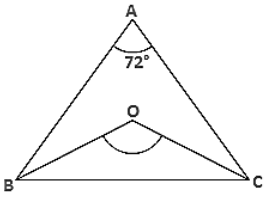Solution:

Given,

ABC is a triangle where ∠A = 72° and the internal bisector of angles B and C meeting O.

In ΔABC,

∠A + ∠B + ∠C = 180°

⇒ 72° + ∠B + ∠C = 180°

⇒ ∠B + ∠C = 180° − 72°

Dividing both sides by '2'

⇒ ∠B/2 + ∠C/2 = 108°/2

⇒ ∠OBC + ∠OCB = 54°

Now, In ΔBOC ⇒ ∠OBC + ∠OCB + ∠BOC = 180°

⇒ 540° + ∠BOC = 180°

⇒ ∠BOC = 180° − 54°=126°

∴  ∠BOC = 126°

Question: 8

The bisectors of base angles of a triangle cannot enclose a right angle in any case.

Solution:

In ΔXYZ,

Sum of all angles of a triangle is 180°

i.e., ∠X + ∠Y + ∠Z = 180°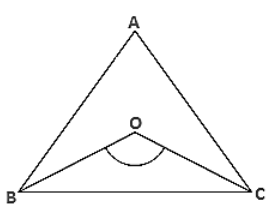Dividing both sides by '2'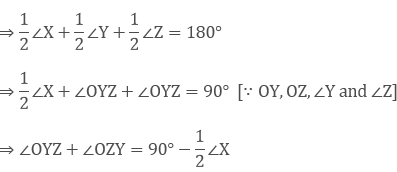Now in ΔYOZ

∴  ∠YOZ + ∠OYZ + ∠OZY = 180°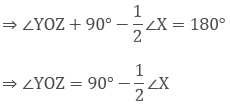Therefore, the bisectors of a base angle cannot enclosure right angle.

Question: 9

If the bisectors of the base angles of a triangle enclose an angle of 135°, prove that the triangle is a right angle.

Solution:

Given the bisectors of the base angles of a triangle enclose an angle of 135°i.e., ∠BOC = 135°

But, We know that⇒ ∠A = 45°(2)

⇒ ∠A = 90°

Therefore, ΔABC is a right angle triangle that is right angled at A.

Question: 10

In a ΔABC, ∠ABC = ∠ACB and the bisectors of ∠ABC and ∠ACB intersect at O such that ∠BOC = 120°. Show that ∠A = ∠B = ∠C = 60°.

Solution:

Given,In ΔABC,

∠ABC = ∠ACB

Dividing both sides by '2'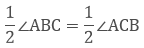⇒ ∠OBC = ∠OCB [ ∴ OB, OC bisects ∠B and ∠C]

Now,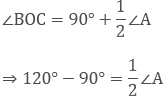⇒ 30°∗ (2) = ∠A

⇒ ∠A = 60°

Now in ΔABC

∠A + ∠ABC + ∠ACB = 180° (Sum of all angles of a triangle)

⇒ 60° + 2∠ABC = 180° [∴ ∠ABC = ∠ACB]

⇒ 2∠ABC = 180° − 60°

⇒ ∠ABC = 120°/2 = 60°

⇒ ∠ABC = ∠ACB

∴ ∠ACB = 60°

Hence Proved.

Question: 11

Can a triangle have:

(i) Two right angles?

(ii) Two obtuse angles?

(iii) Two acute angles?

(iv) All angles more than 60°?

(v) All angles less than 60°?

(vi) All angles equal to 60°?

Solution:

(i) No, Two right angles would up to 180°. So the third angle becomes zero. This is not possible, so a triangle cannot have two right angles. [Since sum of angles in a triangle is 180°]

(ii) No, A triangle can't have 2 obtuse angles. Obtuse angle means more than 90° So that the sum of the two sides will exceed 180° which is not possible. As the sum of all three angles of a triangle is 180°.

(iii) Yes, A triangle can have 2 acute angles. Acute angle means less the 90° angle.

(iv) No, Having angles more than 60° make that sum more than 180°. This is not possible. [Since the sum of all the internal angles of a triangle is 180°]

(v) No, Having all angles less than 60° will make that sum less than 180° which is not possible.[Therefore, the sum of all the internal angles of a triangle is 180°]

(vi) Yes, A triangle can have three angles equal to 60°. Then the sum of three angles equal to the 180°. Such triangles are called as equilateral triangle. [Since, the sum of all the internal angles of a triangle is180°]

Question: 12

If each angle of a triangle is less than the sum of the other two, show that the triangle is acute angled.

Solution:

Given each angle of a triangle less than the sum of the other two

∴ ∠X + ∠Y + ∠Z

⇒ ∠X + ∠X < ∠X + ∠Y + ∠Z

⇒ 2∠X < 180° [Sum of all the angles of a triangle]

⇒ ∠X < 90°

Similarly ∠Y < 90° and ∠Z < 90°

Hence, the triangles are acute angled.
```### Course Features

• 728 Video Lectures
• Revision Notes
• Previous Year Papers
• Mind Map
• Study Planner
• NCERT Solutions
• Discussion Forum
• Test paper with Video Solution